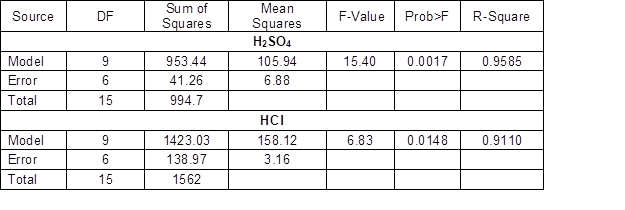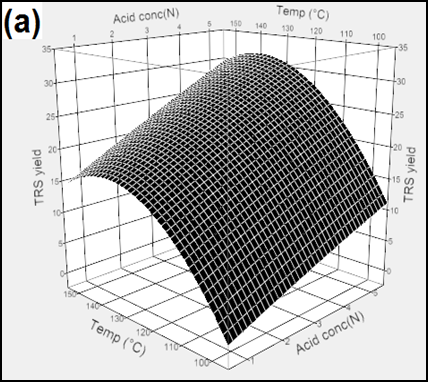Chin, S. X., Chia, C. H., and Zakaria, S. (2013). "Production of reducing sugar from oil palm empty fruit bunch (EFB) cellulose fibres via acid hydrolysis," BioRes. 8(1), 447-460.

#### Abstract

Cellulosic fibre of oil palm empty fruit bunches (EFB) were used as a raw material for acid hydrolysis using mineral acids (H2SO4 and HCl) to produce reducing sugar at moderate temperature and atmospheric pressure. Experiments were carried out to investigate the effect of the hydrolysis parameters, including acid concentration, temperature, and reaction time, on the total reducing sugar (TRS) yield with the aid of response surface methodology (RSM). The preliminary hydrolysis studies of the EFB fibres showed that the presence of lignin in the fibres significantly affected the TRS yield. The maximum predicted TRS yield using H2SO4 was 30.61% under optimal conditions: acid concentration of 5 N, temperature of 139.65 oC, and reaction time of 4.16 h. For the hydrolysis using HCl, the maximum predicted TRS yield is 39.81% under optimal conditions: acid concentration of 4.63 N, temperature of 133.7 oC, reaction time of 2.05 h.

Production of Reducing Sugar from Oil Palm Empty Fruit Bunch (EFB) Cellulose Fibres via Acid Hydrolysis

Siew Xian Chin, Chin Hua Chia,* and Sarani Zakaria

Cellulosic fibre of oil palm empty fruit bunches (EFB) were used as a raw material for acid hydrolysis using mineral acids (H2SO4 and HCl) to produce reducing sugar at moderate temperature and atmospheric pressure. Experiments were carried out to investigate the effect of the hydrolysis parameters, including acid concentration, temperature, and reaction time, on the total reducing sugar (TRS) yield with the aid of response surface methodology (RSM). The preliminary hydrolysis studies of the EFB fibres showed that the presence of lignin in the fibres significantly affected the TRS yield. The maximum predicted TRS yield using H2SO4 was 30.61% under optimal conditions: acid concentration of 5 N, temperature of 139.65 oC, and reaction time of 4.16 h. For the hydrolysis using HCl, the maximum predicted TRS yield is 39.81% under optimal conditions: acid concentration of 4.63 N, temperature of 133.7 oC, reaction time of 2.05 h.

Keywords: Acid catalyst; Glucose; Response surface methodology; Total reducing sugar

Contact information: School of Applied Physics, Faculty of Science and Technology, Universiti Kebangsaan Malaysia, 43000 Bangi, Selangor, Malaysia; *Corresponding author: chiachinhua@yahoo.com

INTRODUCTION

On a worldwide level, energy demand is growing constantly due to the progressive exhaustion of fossil and non-renewable energy resources (Folkedahl et al. 2011). Among renewable energy resources, lignocellulosic biomass constitutes a major class of carbon-neutral energy resources, which can be used to produce fine chemicals and bio-fuels. Additionally, the utilization of lignocellulosic biomass as chemical feedstock can also decrease dependence on fossil resources (Morales-delaRosa et al. 2012). Lignocellulosic biomass can be obtained from various agricultural by-products, such as bagasse, rice husk, palm oil waste, logging residues, rice straw, and coconut shells. These by-products in large quantities have created various environmental problems, such as the harmful effects of open burning and disposal of the by-products (Carlos and Ba Khang 2008). Malaysia is the second largest palm oil producer in the world. Generally, palm oil industries in Malaysia produce over 11.9 million tons of palm oil per year. Meanwhile, about 100 million tons of biomass residues are produced every year. This large amount of residue includes oil palm trunks, fronds, kernel shells, press fruit fibre, and empty fruit bunches (EFB) (Abdul Khalil et al. 2010).

In Malaysia, about 17 million tons of oil palm EFB is produced from the palm oil extraction process every year. Oil palm EFB fibres are composed of about 44.2% cellulose, 33.5% hemicellulose, and 20.4% lignin (Hamzah et al. 2011). With such an abundance of valuable and renewable natural components, several approaches have been developed to utilize oil palm EFB fibres to produce other materials, including paper pulp (Jiménez et al.2009), thermoset polymer (Chai et al. 2009), composite boards (Khalid et al. 2008), and activated carbon (Alam et al. 2009).

In the last decade, many studies have utilized lignocellulosic biomass to produce fine chemicals, such as glucose, furfural, hydroxymethyl furfural, levulinic acid, etc. (Thomsen et al. 2009). Oil palm EFB fibres have been used to produce xylose using acid hydrolysis (Zhang et al. 2012). Meanwhile, there have also been studies on the production of glucose from oil palm EFB fibres using enzymatic saccharification, which requires a long reaction time, ranging from 24 h to 72 h (Hamzah et al. 2011; Shamsudin et al. 2012). On the other hand, hydrolysis using mineral acids, such as HCl and H2SO4, has been widely reported due to their high efficiency and low production cost (Li et al. 2008; Laopaiboon et al. 2010; Hu et al. 2010).

As mentioned above, EFB fibres are comprised of 20.4% lignin. Lignin holds an integral part in the cell wall, while cellulose and hemicellulose are strongly bonded with lignin to form rigid cell wall structure. Hence, pretreatment is important for removing the lignin, which acts as a barrier for acid hydrolysis or enzymatic attack (Mosier et al. 2005; Sannigrahi et al. 2008).

In the present study, oil palm EFB fibres were used as raw material to produce reducing sugar. Prior to the optimization studies, the effect of lignin content in the EFB fibres on the hydrolysis efficiency using HCl and H2SO4 was investigated. This is critical because lignin is one of the major components in most lignocellulosic biomass. In order to further investigate the viability of EFB cellulose fibres for producing reducing sugar, optimization of the hydrolysis reactions under moderate temperature and atmospheric pressure were carried out with various experimental parameters using response surface methodology (RSM).

EXPERIMENTAL

Chemicals and Materials

Analytical grade chemicals, including sulfuric acid (H2SO4), hydrochloric acid (HCl), sodium hydroxide (NaOH), sodium hypochlorite (NaClO2), acetic acid (CH3COOH), and glucose were purchased from Sigma Aldrich. Oil palm EFB fibres obtained from Szetech Engineering Sdn. Bhd. were washed and ground before being sieved into fibres with dimensions between 1 and 4 mm.

Methods

Pretreatment and characterizations of EFB fibres

The raw EFB sample (Sample A) was subjected to several pre-treatment processes, including alkaline and bleaching treatments, for preparing EFB fibre samples with different lignin contents (Sample B and C). Samples B was obtained via one cycle of alkaline-bleaching treatment. The alkaline treatment was carried out using 4wt% NaOH at 70 ͦ C for 2 hours, while the bleaching process was carried out using 0.18 M of NaClO2 in CH3COOH buffer solution at 70 ͦ C for 1 h (Hamzah et al. 2011). Sample C was obtained by three cycles of the alkaline-bleaching process. The lignin content of the three EFB samples was determined according to the TAPPI method (Piarpuzán et al. 2011). The morphology of the EFB fibre samples was examined using a scanning electron microscope (SEM – Philips XL-30).

Powder X-ray diffractions of Sample A, Sample B, and Sample C were obtained using a Bruker AXS D8 Advance instrument. The samples were scanned in the range of 5 to 60. The degrees of crystallinity (CrI%) of the EFB samples were determined using Segal’s method (Segal et al. 1959, Park et al. 2010).

Preliminary acid hydrolysis using HCl and H2SO4

Acid hydrolysis of Sample A, Sample B, and Sample C was performed using an Orbit system (Syrris). Each EFB sample (0.6 g) was hydrolyzed using 25 mL of 5 N HCl and 5 N H2SO4 separately in a reaction tube at 150 C for 2 h. The temperature of the reaction was monitored with a thermocouple. The reaction was performed under atmospheric pressure and stirred using a magnetic stirrer. After the hydrolysis reaction, the reaction tube was quickly immersed in an ice water bath. The hydrolyzate was then centrifuged at 12,000 rpm for 15 min to separate the solid and liquid phases. The obtained solution was then neutralized with 1 N NaOH solution. The total reducing sugar (TRS) in the hydrolyzate was determined using the 3,5-dinitrosalicyclic acid (DNS) method (Zhang et al. 2012). Briefly, the hydrolyzate (3 mL) was mixed with a DNS reagent (3 mL) in a test tube and incubated in a water bath at 90 oC for 10 min. The color intensity of the mixture was measured using a UV-Vis spectrophotometer (Jenway 7315) at a wavelength of 540 nm (Amarasekara and Wiredu 2012). The concentration of the TRS was calculated based on a standard curve obtained from glucose with various concentrations (R= 0.9998).

Acid hydrolysis of EFB cellulose fibre

The EFB cellulose fibre (Sample C) was selected for further hydrolysis optimization studies. This is based on the results obtained from a preliminary study in which Sample C was found to give a higher TRS yield as compared with samples A and B. Different reaction parameters were selected for optimization purposes, including reaction temperature (oC), reaction time (h), and acid concentration (N). The TRS yield was determined using the DNS method as stated above. The morphology and the X-ray diffraction pattern of the EFB cellulose fibre residues after acid hydrolysis were examined. In this study, two types of acids, HCl and H2SO4 were used as catalysts for the hydrolysis of the EFB cellulose fibre.

Experimental design of RSM (optimization of EFB cellulose hydrolysis)

Central composite face-centred (CCF) experimental design was performed to obtain the optimized parameters for obtaining the highest TRS yield. The design consisted of 16 experiments with two replication center points.

In this work, there were three variables: acid concentration, temperature, and time. The effects of the X1 (acid concentration, A), X(temperature, T), andX3 (time, t) were tested at three variables levels in the acid hydrolysis reaction (Table 1). For statistical calculation, the variables were coded according to Equation (1).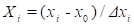(1)

where Xi is the coded value for the independent variable, xi is the real value of the independent variable, x0 is the real value of the independent variables on the center point, and Δ xi is the step change value. The specific codes are shown in Equation (2), (3) and (4).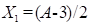(2)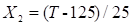(3)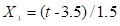(4)

A polynomial equation model for the predicted response of TRS yield (Yi) was then developed, as shown in Equation 1, where βis the offset term, β1β2,and β3 are the linear effect terms, β11β22, and β33 are the squared effects, and β12β13, and β23 are the interaction effects. This polynomial equation is intended to describe the relationship between the independent variables and the response variables. The significance of each equation coefficient was determined by P-values. Meanwhile, the fitted polynomial equation was expressed by surface plots in order to visualize the relationship between the independent variables and the response variables to deduce optimum conditions for this acid hydrolysis reaction (Chang et al. 2007)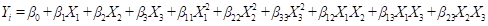(5)

Table 1. Parameter Levels for the Three Factors in the Acid Hydrolysis Reaction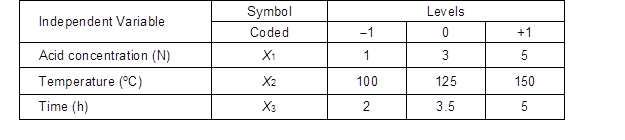RESULTS AND DISCUSSION

Pretreatment of EFB Fibres

Figure 1 shows images of the raw EFB fibres and the EFB fibres after pretreat-ment. It can be seen that the fibres became brighter after the pretreatment process, which can be attributed to the removal of lignin (Table 2). The lignin content of the raw EFB fibres was 21.27 w/w %, which decreased to 5.34 w/w % and 0.23 w/w % after one and three cycles of pretreatment, respectively.

The morphology of the EFB fibre samples is presented in Fig. 2. It is seen that prior to pretreatment, the physical structure of the raw EFB fibres was rigid with tightly packed cellulose micro fibrils. It can also be observed that there were many cavities on the surface of the raw EFB fibres, which is potentially due to the detachment of silica. It was reported previously that the oil palm EFB fibres contain a high level of silica (Hamzah et al. 2011; Kargbo et al.2009; Shinoj et al. 2011).

Figure 2(b) shows the SEM image of the EFB fibres after one pretreatment cycle. It can be seen that the original bundled structure of the raw EFB fibres was broken into single fibres. After three cycles of pretreatment (Fig. 2c), the surface of the EFB fibres became rougher, and the existence of microfibrils can be clearly seen as compared to Fig. 2(b).

The diffraction patterns of the EFB fibre samples are presented in Fig. 3. All samples exhibited a crystalline pattern with a diffraction peak between 16 and 23, representing the typical crystal structure of cellulose I. There were no obvious changes in terms of the diffraction pattern of the EFB fibre samples (Table 2), suggesting that the pretreatment process did not affect the crystal structure of the EFB fibres; however, there was a slight increase in the degree of crystallinity (Table 2) of the fibres after the pretreatment processes due to the removal of amorphous fractions (hemicellulose and lignin) from the fibres (Li et al. 2011).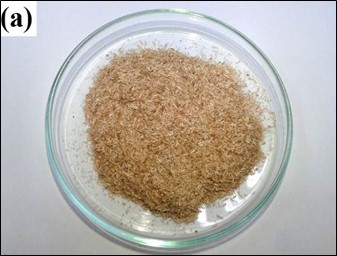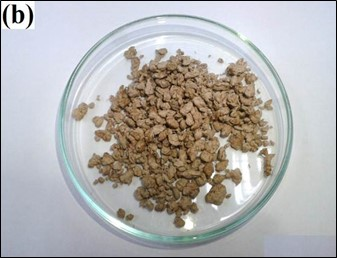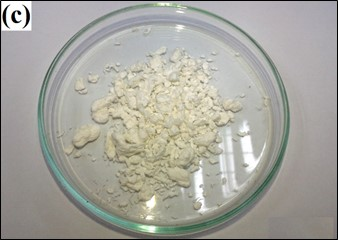Fig. 1. Oil palm EFB fibres: (a) Sample A – raw EFB fibres, (b) Sample B – one cycle pre-treated EFB fibres, and (c) Sample C – three-cycle pre-treated EFB fibres

Table 2. Lignin Content and Degree of Crystallinity (CrI%) of EFB Fibre Samples (A-C)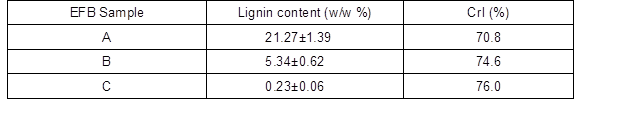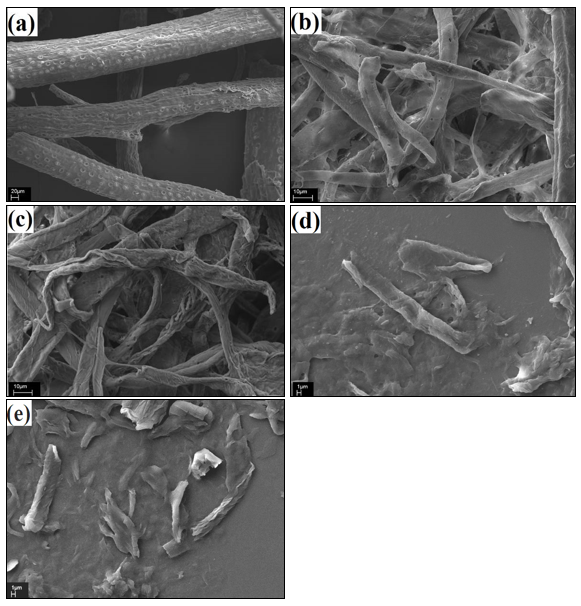Fig. 2. SEM micrographs of EFB fibre samples: (a) Sample A, (b) Sample B, (c) Sample C, (d) residue of EFB cellulose fibre after H2SO4 hydrolysis, and (e) residue of EFB cellulose fibres after HCl hydrolysis

Acid Hydrolysis of the EFB Fibers

Figure 4 shows the TRS yields obtained from the hydrolysis of EFB fibre samples (A, B, and C) using 5 N H2SO4 and 5 N HCl at 150 C for 2 h. Generally, the TRS produced from Sample C was higher than that of Sample A and Sample B. This can be attributed to the lower lignin content of the EFB fibres. The presence of lignin may serve as a barrier for the penetration of the acid into the inner part of the EFB fibres. The study also found that lignin will precipitate on cellulose during hydrolysis and thus retard the reaction (Sasaki et al. 2012). It can also be observed that the degree of crystallinity of the EFB fibres did not contribute much on the TRS yield. Figures 2(d) and (e) show the SEM micrographs of the residue of EFB cellulose fibres (Sample C) after hydrolysis using 1 N H2SO4 and 1 N HCl for 2 h, respectively. It was clearly shown that the EFB cellulose fibre was hydrolyzed into smaller fragments and no longer retained the original fibrous structure. The XRD results presented in Fig. 3 show that the crystal structure of the EFB cellulose fibre was maintained after hydrolysis, while the degree of crystallinity of the EFB cellulose residues was increased from 76% (Table 2) to about 82%. This can further confirm that the acid hydrolysis pathway begins at an amorphous crystalline cellulose region with less order (Sannigrahi et al.2008).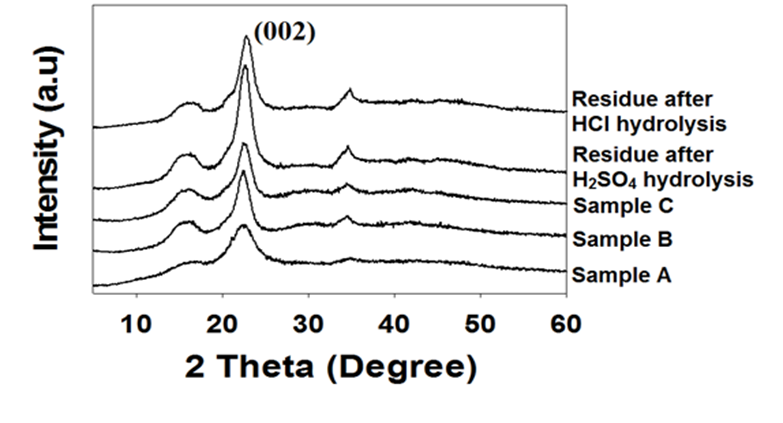Fig. 3. XRD patterns of EFB fibres samples (A-C) and EFB cellulose residue after acid hydrolysis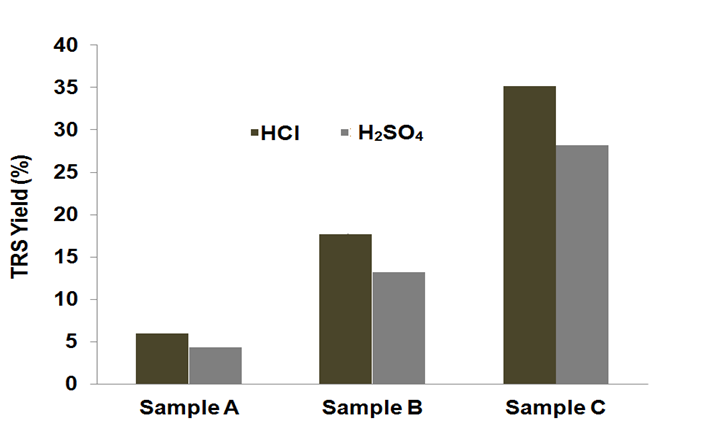Fig. 4. TRS yield of Sample (A-C) obtained from acid hydrolysis using H2SO4 and HCl

Optimization of Acid Hydrolysis of EFB Cellulose

Hydrolysis of EFB fibre cellulose using H2SO4

Table 3 shows the relationship between the three independent variables (acid concentration, temperature, and time) and the response (TRS yield) for the hydrolysis using H2SO4.

A quadratic model was fitted to the experimental data and a new formula for the TRS yield was obtained as shown in Eq. 6,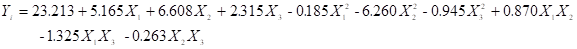(6)

where Yis the response, and X1X2, and Xare the coded values of acid concentration, temperature, and time, respectively. The coefficients of Equation 6 were calculated using RSM and the values estimated are listed in Table 4. The estimated parameters show the coefficients and significance of the linear, cross product, and quadratic terms on each response. It can also be seen that all the main effects and quadratic effect of temperature are statistically significant according to the P-value (P < 0.05). The function of this P-value is to check the significance of each of the coefficients and to identify the pattern of mutual interactions between all the independent variables (Chang et al. 2007; Karthikeyan et al. 1996; Yemiş and Mazza 2012). This means that acid concentration, temperature, and time are directly related to the conversion of EFB cellulose fibres to TRS.

Significance testing on the regression models, which focused on the relationship between a dependent variable and independent variables, was performed using analysis of variance (ANOVA), and the results are presented in Table 5. The ANOVA with a low probability 0.0017 shows that the fitted model is highly significant. The coefficient of determination (R2), which demonstrates the agreement between the observed and predicted results, was used to check the quality of fit of the equation. Rhas been suggested to be at least 0.80 for a good fit of a model (Hsu 1995; Yemiş and Mazza 2012). The R2obtained was 95.9 %, suggesting a good fitting of the quadratic model.

The predicted optimal conditions for the hydrolysis of EFB cellulose fibre using H2SO4 was obtained based on the regression equation as illustrated by the 3D response surface plots in Fig. 5. The maximum predicted TRS yield was also indicated in the 3D response surface plots. The TRS yield increased linearly with the increase of H2SO4 concentration. As seen in Fig. 5(a) and Fig. 5(b), higher H2SOconcentration enhanced the TRS yield. In this work, the highest TRS yield was achieved using 5 N H2SO4 while incorporating other experimental conditions.

Figures 5(a) and (c) show the effect of reaction temperature on the TRS yield. Initially, TRS yield increased linearly with temperature. The TRS yield achieved a maximum value at 139.65 oC and decreased with further temperature increment. Theoretically, higher temperature could accelerate the rate of cellulose hydrolysis to TRS; however, unwanted side reaction products could also be produced, such as hydroxymethylfurfural, furfural, levulinic acid, and acetic acid, at elevated temperature (Amarasekara and Wiredu 2012; Chang et al. 2007; Li et al. 2008).

In Figs. 5(b) and (c), time shows a relative significant interaction with other variables (acid concentration and temperature). The TRS yield increased as the reaction time increased. In this work, the maximum TRS yield was achieved with a reaction time of 4.16 h; however, further increase in the reaction time resulted in a lower TRS yield, which might be due to the decomposition of TRS to form hydroxymethylfurfural, furfural, levulinic acid, and acetic acid (Chang et al. 2007; Li et al. 2008) in the presence of acid. Basically, the acid concentration and temperature significantly affect the time required for the overall hydrolysis of EFB cellulose fibres using H2SO4.

Based on the 3D response surface plots, the optimum parameter to achieve the highest TRS yield using H2SO4 are an acid concentration of 5 N, a reaction temperature of 139.65 oC, and a reaction time of 4.16 h. The predicted maximum TRS yield was calculated to be (30.61% or 1.81 g/L). A confirmation run, based on the predicted optimum conditions, was performed to verify the stability of the model. The TRS yield was 29.99%, which is close to the predicted value.

Table 3. Experimental Design and Results of the TRS Yields Obtained from the Hydrolysis of EFB Cellulose Fibres using H2SO4 and HCl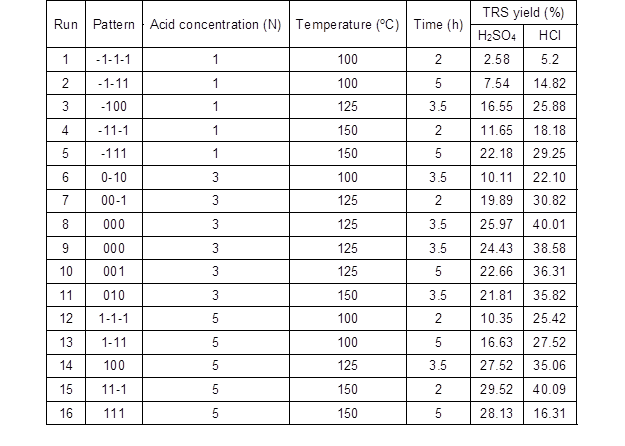Table 4. Significance of Regression Coefficient of TRS Yields for the Hydrolysis Using H2SO4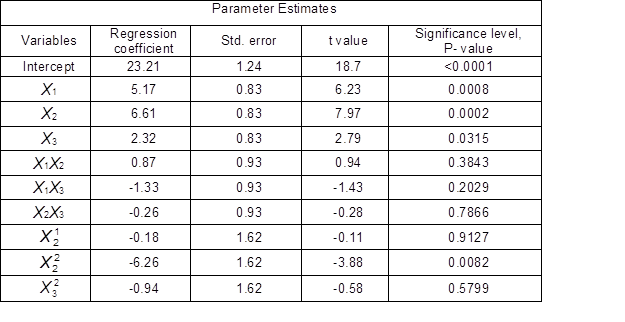Table 5. Analysis of Variance (ANOVA)